Area Effects

An area effect originates from a single square or creature. Unless otherwise stated, an area effect affects [Flying], [Swimming], and [Burrowing] creatures the same way it affects creatures without those conditions. Unless otherwise stated, multiple instances of an area effect affect those within them separately. For example, if two effects each deal damage to all within a created spread, creatures that happen to be within the spreads created by both effects would take damage from both effects.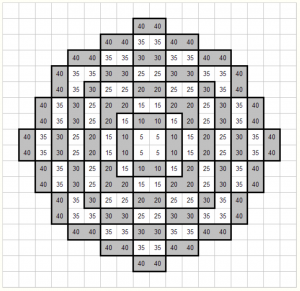The listed numbers are in feet; consult them to determine if your spread would reach that far.

Spreads expand evenly in all directions. If a spread originates from a square, its radius is measured from a vertex of that square. (The source of the effect that creates the spread chooses the vertex.) If a spread originates from a creature, its radius is measured from each edge of each square that creature occupies, and its area includes each square within any of these measurements. (A spread that originates from a creature that occupies more than one square is larger than an identical spread originating from a creature that occupies only a single square.)

Wedges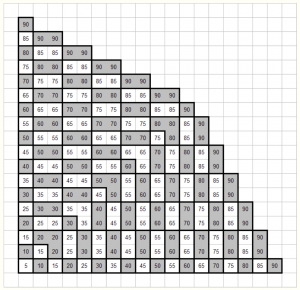The listed numbers are in feet; consult them to determine if your wedge would reach that far.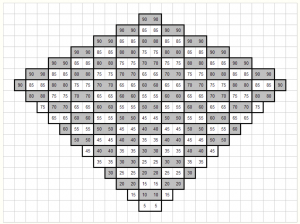The listed numbers are in feet; consult them to determine if your wedge would reach that far.

Wedges expand from a vertex of a square in a quarter-circle out to their given length. If a wedge originates from a square, the source of the effect chooses a vertex of that square. If a wedge originates from a creature, the source of the effect chooses a square that creature occupies and chooses a vertex of that square. A wedge can point in any of eight directions when drawn on a grid (up and down, sideways, and diagonally).

Drawing Wedges

Wedges come in two varieties: diagonal wedges and orthogonal wedges. Wedges are determined as follows:

1. If the wedge originates from a square, the source of the effect chooses a vertex of that square. If the wedge originates from a creature, the source of the effect chooses a square that creature occupies and chooses a vertex of that square.
2. The source of the effect chooses one non-diagonal direction, then chooses either one other non-diagonal direction that is not opposite the first chosen direction or each other non-diagonal direction that is not opposite the first chosen direction.
3. The area of a wedge includes each square within a distance equal to the wedge's length of the vertex chosen in Step 1 that is two of the directions chosen in Step 2 from that vertex. If more than two directions were chosen in Step 2, the area of the wedge includes only squares that are no less distance in the first direction chosen in Step 2 than in any other direction chosen in Step 2 from that vertex.

Lines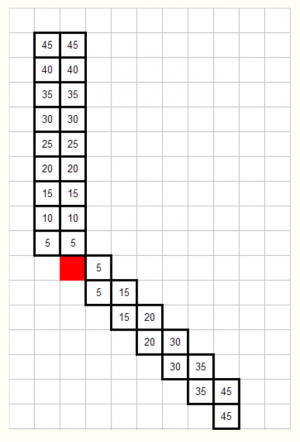The listed numbers are in feet; consult them to determine if your line would reach that far.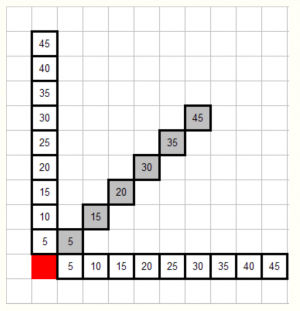The listed numbers are in feet; consult them to determine if your line would reach that far.

Lines extend in a given direction. This direction is chosen by the source of the effect, unless otherwise stated. If a line originates from a square, it extends from that square. If a line originates from a creature, it extends from a square that creature occupies (the source of the effect chooses this square). Unless otherwise stated, a line is 5 ft wide.

Drawing Lines

Lines are determined as follows:

1. If the line originates from a square, the source of the effect chooses that square. If the line originates from a creature, the source of the effect chooses a square that creature occupies.
2. The source of the effect chooses one non-diagonal direction. The source of the effect may then choose another non-diagonal direction that is not opposite the first chosen direction.
3. The source of the effect chooses one non-diagonal direction. If only one direction was chosen in Step 2, this direction cannot be the same as the direction chosen in Step 2 or the direction opposite that direction. (Otherwise this direction may be the same as a direction chosen in Step 2.)
4. The area of a line includes the following:
1. Each square within a distance equal to the line's length of the square chosen in Step 1 that is each of the directions chosen in Step 2 from that square and is equal distance in each of the directions chosen in Step 2 as in each other direction chosen in Step 2 from that square. If only one direction was chosen in Step 2, each square must be in the direction chosen in Step 2 and no other direction from the square.
2. Each square within a distance equal to half the line's width of any square from Step 4.1 that is the direction chosen in Step 3 and no other direction from that square, and each square within a distance equal to half the difference of the line's width and 5 ft of any square from Step 4a that is the direction opposite the direction chosen in Step 3 and no other direction from that square.